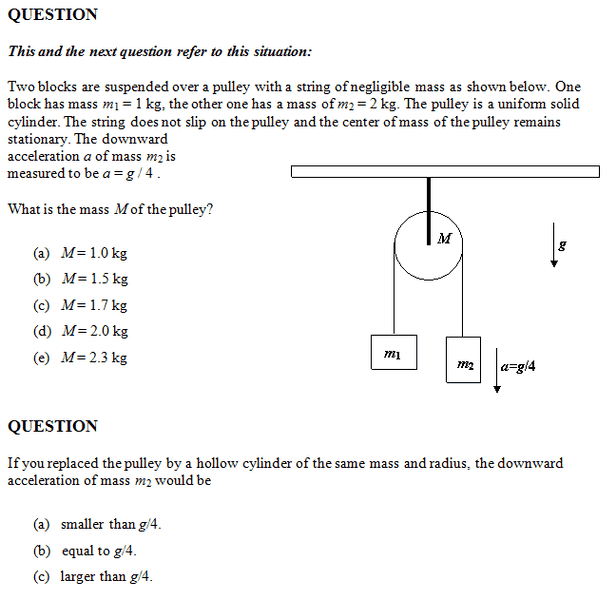# Two blocks and a Pulley

## Homework Statement

http://imgur.com/MaT4NTorque = Fr

## The Attempt at a Solution

1) I tried
m2 g r - m1 g r = 1/2 M r^2 * (acceleration / r)
didn't work

2)I tried replace the moment of inertia equation to above equation,still didn't work

#### Attachments

Doc Al
Mentor
1) I tried
m2 g r - m1 g r = 1/2 M r^2 * (acceleration / r)
didn't work
You cannot assume that the tension in the ropes equals the weight of the blocks. If that were true, the masses would be in equilibrium, not accelerating.

Instead, apply Newton's 2nd law to each block and to the pulley. Combine those three equations to solve for the unknowns.

Hi!
I tried this m2gR - m1gr +mgR =0

I am confused about which forces has torque on this problem?
How do I suppose to set up with an equation with both torque and Newton's second law?
Thanks!

Also,I am still confused about second part. Thanks

Doc Al
Mentor
Hi!
I tried this m2gR - m1gr +mgR =0
Not sure what you're doing here.
I am confused about which forces has torque on this problem?
Torque is only relevant for the pulley. The forces creating the torque on the pulley are the rope tensions on each side of the pulley.
How do I suppose to set up with an equation with both torque and Newton's second law?
For the two blocks, you'd use the usual form of Newton's 2nd law: ΣF = ma

But for the pulley, you'll need the rotational form, which uses torque, rotational inertia, and angular acceleration: ΣTorque = I*alpha.

Also,I am still confused about second part. Thanks
To solve the second part, you'll need to replace the rotational inertia of the pulley with that of a hollow cylinder. Then you'd have to determine whether that causes the acceleration to increase, decrease, or remain the same. (Hint: How does the rotational inertia of a hollow cylinder compare to that of solid cylinder?)